# Affine variety

(diff) ← Older revision | Latest revision (diff) | Newer revision → (diff)

affine algebraic variety

A generalization of the concept of an affine algebraic set. An affine variety is a reduced affine schemeof finite type over a field, i.e., whereis a commutative-algebra of finite type without nilpotent elements. The affine variety, whereis the ring of polynomials over, is called affine space overand is denoted by. An affine scheme is an affine variety if and only if it is isomorphic to a reduced closed subscheme of an affine space. Each system of generatorsof a-algebradefines a surjective homomorphism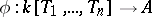, defined by the formula. Letbe the algebraic closure of. The subset of the setconsisting of the common zeros of all the polynomials of the ideal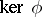is an affine algebraic set over. The coordinate ring of such an affine algebraic set is isomorphic to the ring. Each affine algebraic set overin turn defines an algebraic variety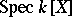, where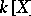is the coordinate ring of. The set of points of an affine variety is in a one-to-one correspondence with the irreducible subvarieties of the corresponding affine algebraic set.

To each affine variety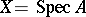a functor on the category of-algebras is assigned. It is defined by the correspondence: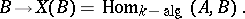If(respectively, if), the elements of the set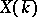(respectively,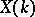) are called geometric (respectively, rational) points of. The setis in a bijective correspondence with the set of maximal idealsof the ring, and with the set of points of an algebraic setwhose coordinate ring is isomorphic to. The spectral topology in the spaceinduces on the everywhere-dense subset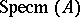a topology which corresponds to the Zariski topology on.